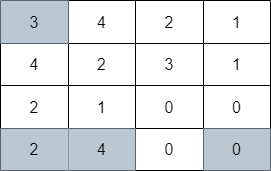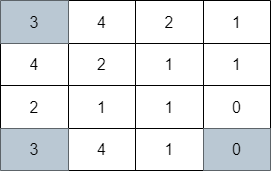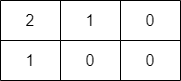2617. Minimum Number of Visited Cells in a Grid
Hard
264
25

You are given a 0-indexed `m x n` integer matrix `grid`. Your initial position is at the top-left cell `(0, 0)`.

Starting from the cell `(i, j)`, you can move to one of the following cells:

• Cells `(i, k)` with `j < k <= grid[i][j] + j` (rightward movement), or
• Cells `(k, j)` with `i < k <= grid[i][j] + i` (downward movement).

Return the minimum number of cells you need to visit to reach the bottom-right cell `(m - 1, n - 1)`. If there is no valid path, return `-1`.

Example 1:```Input: grid = [[3,4,2,1],[4,2,3,1],[2,1,0,0],[2,4,0,0]]
Output: 4
Explanation: The image above shows one of the paths that visits exactly 4 cells.
```

Example 2:```Input: grid = [[3,4,2,1],[4,2,1,1],[2,1,1,0],[3,4,1,0]]
Output: 3
Explanation: The image above shows one of the paths that visits exactly 3 cells.
```

Example 3:```Input: grid = [[2,1,0],[1,0,0]]
Output: -1
Explanation: It can be proven that no path exists.
```

Constraints:

• `m == grid.length`
• `n == grid[i].length`
• `1 <= m, n <= 105`
• `1 <= m * n <= 105`
• `0 <= grid[i][j] < m * n`
• `grid[m - 1][n - 1] == 0`
Accepted
6.1K
Submissions
22.2K
Acceptance Rate
27.7%

Seen this question in a real interview before?
1/4
Yes
No

Discussion (0)

Related Topics